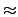Next: 61-bit Prime Modulus Linear Up: Random Number Generators Previous: 48-bit Linear Congruential Generator

## 48-bit CRI Linear Congruential Generator

This algorithm is provided by the operating system on computers manufactured by Cray Research Incorporated (CRI), and as such it has been heavily used at computer centers employing CRI hardware. We provide it in order to support comparison with the other random number generators provided in the RNG library. This generator suffers from the same patterns in the low order bits as the 64-bit generator, but these patterns become more significant because of the smaller width of the random number state. The next value for the random number state, x[n], is determined from the current value, x[n - 1], by

 x[n] = a x[n - 1]   (mod248)   . (5)
The value of the multiplier, a = 44485709377909, is hardwired into the implementation and is known to produce a good random number list (note that lcg48 and lcgCRI have the same multiplier). Both the multiplier, and the random number state are odd. The period of this random number generator is 2467.0 x 1013 per stream. The generator actually creates two independent streams based on the value of the "twos" bit in the random number state that is preserved by the recurrence relation. The hash functions associated with this generator set the "twos" bit randomly, taking full advantage of the pair of random number streams produced by the recurrence relation. The value zero does not occur for this random number generator. The interface routines for this generator are: Rng_Type RngCRISeed( Rng_UInt32 i, Rng_UInt32 j );

Rng_Type RngCRISpawn( Rng_Type *x );

int iRngCRI( Rng_Type *x );

double dRngCRI( Rng_Type *x );

float fRngCRI( Rng_Type *x );

The pattern content of this generator is frightening, making it somewhat amazing that it has been successfully used for so many years. Because this generator duplicates the arithmetic of a commonly used random number generator, and a zeroed state is trapped, it can be useful during the debugging stage of program transformation to a per-particle state approach. Past its utility for debugging purposes, our advice is to only use this generator to check application sensitivity to the quality of the random number stream.Next: 61-bit Prime Modulus Linear Up: Random Number Generators Previous: 48-bit Linear Congruential Generator